## 流动性基本介绍

uniswap流动性是指交易pool池中的代币，由流动性提供者存入到池中，我们之前说的的价格变化就是基于流动性数量来计算的。

## 添加流动性

Uniswap用一个三元组（e, t, l)来代表交易平台的状态，其中e代表ETH的数量，t代表其他Token的数量，l代表当前流动性总量。添加流动性导致的状态转移方程如下：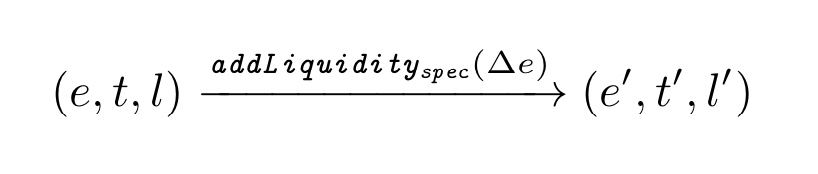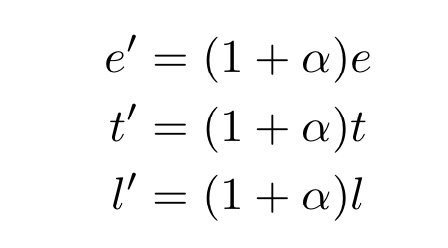$\alpha =\Delta e/e$ , 流动性提供者即投资者可以存入 ∆e数量的 ETH 和 ∆t = t′ − t 的其他token，来挖取∆l = l′ − l 流动性。

1. e : t : l = e′ : t′ : l′

2. k < k′

3. $\frac{k'}{k}=(\frac{l'}{l})^2$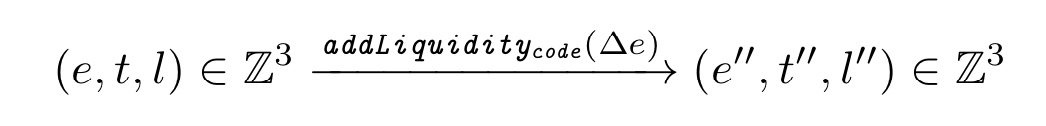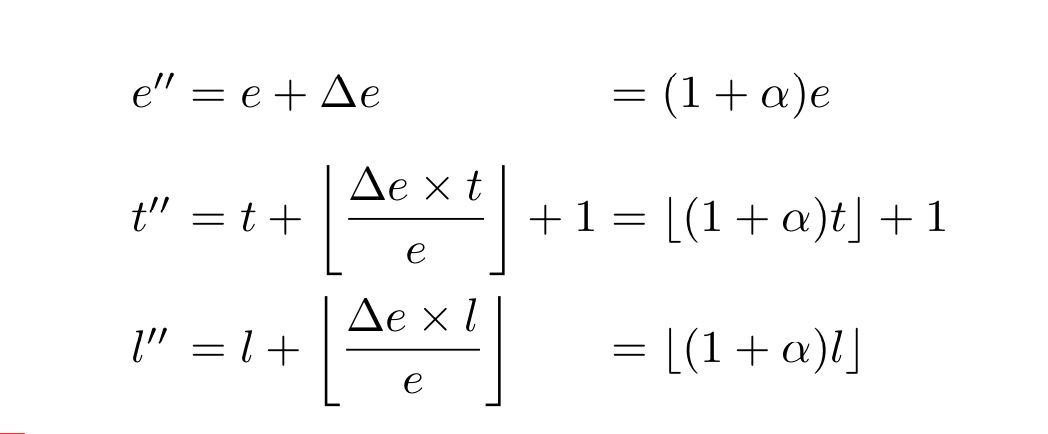t'', l''计算中，采用了向下取整，考虑到具体计算过程中精度的因素，尤其以太坊虚机整数计算的问题。

## 移除流动性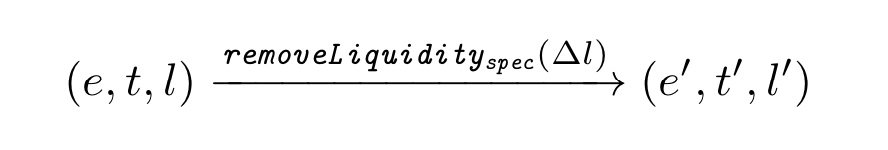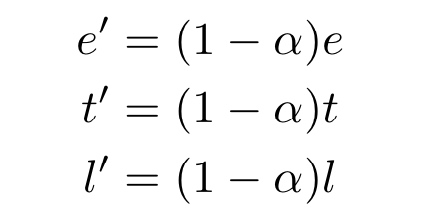$\alpha =\Delta l/l$, 表示流动性提供者销毁∆l的流动性，得到∆e = e − e′ 个ETH和∆t = t − t′ token 对于k = e × t，k′ = e′ × t′ 可以得到：

1. e : t : l = e′ : t′ : l′

2. k > k′

3. $\frac{k'}{k}=(\frac{l'}{l})^2$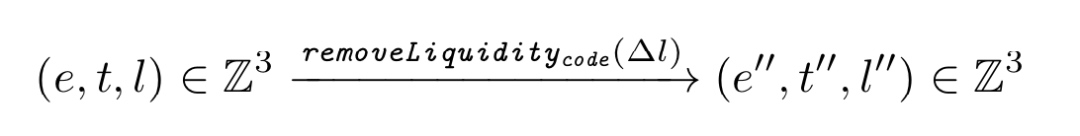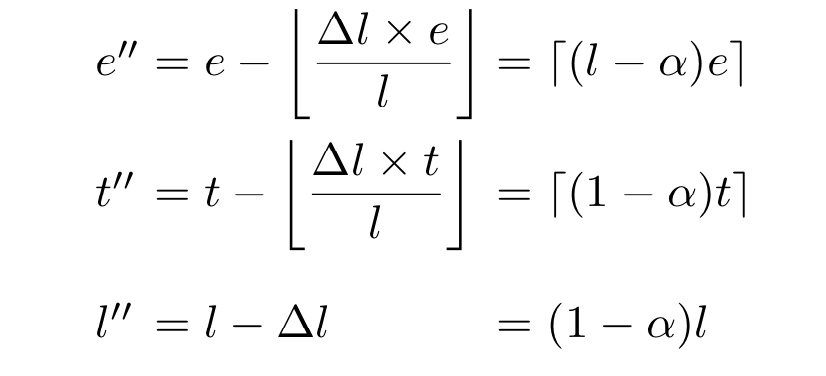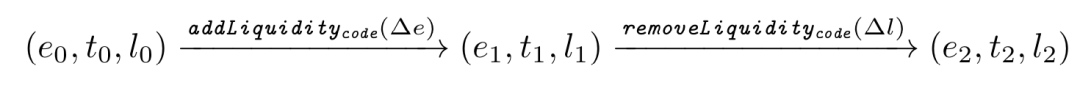1. $e_0<e_2$

2. $t_0<t_2$

3. $l_0<l_2$

## 小结

• 发表于 2020-09-26 14:25
• 阅读 ( 785 )
• 学分 ( 128 )
• 分类：入门/理论